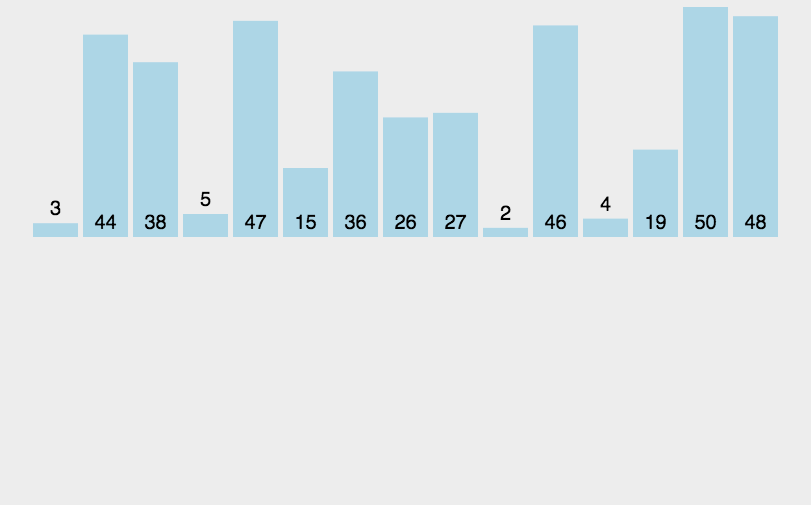# 归并排序（Merge Sort）

## 算法描述

• 把长度为n的输入序列分成两个长度为n/2的子序列；
• 对这两个子序列分别采用归并排序；
• 将两个排序好的子序列合并成一个最终的排序序列。

## 动图演示## 代码实现

public static void main(String[] args) {
int[] array = {3, 44, 38, 5, 47, 15, 36, 26, 27, 2, 46, 4, 19, 50, 48};
// 只需要修改成对应的方法名就可以了
mergeSort(array);

System.out.println(Arrays.toString(array));
}

/**
* Description: 归并排序
*
* @param array
* @return void
* @author JourWon
* @date 2019/7/11 23:37
*/
public static void mergeSort(int[] array) {
if (array == null || array.length <= 1) {
return;
}

sort(array, 0, array.length - 1);
}

private static void sort(int[] array, int left, int right) {
if (left == right) {
return;
}
int mid = left + ((right - left) >> 1);
// 对左侧子序列进行递归排序
sort(array, left, mid);
// 对右侧子序列进行递归排序
sort(array, mid + 1, right);
// 合并
merge(array, left, mid, right);
}

private static void merge(int[] array, int left, int mid, int right) {
int[] temp = new int[right - left + 1];
int i = 0;
int p1 = left;
int p2 = mid + 1;
// 比较左右两部分的元素，哪个小，把那个元素填入temp中
while (p1 <= mid && p2 <= right) {
temp[i++] = array[p1] < array[p2] ? array[p1++] : array[p2++];
}
// 上面的循环退出后，把剩余的元素依次填入到temp中
// 以下两个while只有一个会执行
while (p1 <= mid) {
temp[i++] = array[p1++];
}
while (p2 <= right) {
temp[i++] = array[p2++];
}
// 把最终的排序的结果复制给原数组
for (i = 0; i < temp.length; i++) {
array[left + i] = temp[i];
}
}


## 算法分析

07-27228807-18769
09-282488
03-251万+
08-217245
08-1134万+
04-271000
08-031万+
04-247944